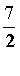# UMB Practice Test C

The questions belowwill review the areas of mathematics that are covered in Test C. These areasare:

* slopes and intercepts of straightlines

* parabolas and quadratic equations

* exponential, logarithmic and trigonometric functions: their graphs and inversefunctions

Youshould try to review these questions (and take Test C) if you have successfullycompleted an Intermediate Algebra course with Trigonometry. Successful completion of TestC will place you into Calculus (Math 140) and Introduction to ComputerProgramming (CS 110). After submitting the review questions youwill receive an analysis of what courses you are eligible to take if this reviewhad been TestC instead of Practice Test C. In addition, a link will be providedto an interactive PreCalculus review which might be helpful for any additionalreview.

In each question below you should restrict the values of any letters to those valuesthat make all expressions well-defined and equal to real numbers. There are 43questions.

Click the Submitbutton at the bottom of the test when you are finished.

Please forward any comments, criticisms, suggestions to: Michael Greeley

Part One
In problems 1 - 4, perform any indicated operations, then simplify: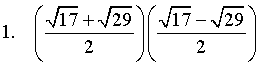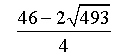11.5 -12 -6 -3
 2 x - 510 æçè 1x - 5 - 1x + 5 ö÷ø

 x - 5x
 - xx2 - 25
 - 1x - 5
0
 1x + 5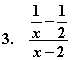- 12x
-1 x
 12
(2 - x)(x - 2)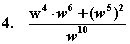w24 + 1 1 + w10 w14 + 1 w10 2

5.  Which of these inequalities is equivalent to |x - 2| < 1?

 x < 3 x < -1 -1 < x 1 < x < 3 -3 < x < -1

6.  If a is a real number such that a3 = a, which of the following mustbe true about a?

 a is positive a is negative a = -1 a = -1, a = 0, or a = 1 a is between -1 and 0

7.  Line L passes through the point (-1, 10) and has slope equal to -7.Find an equation of the line that passes through thepoint (-1, 10) and isperpendicular to line L.

 7y - x = 71 y = -7x + 3 y = -x + 10 y = 7x - 3 none of these.

8.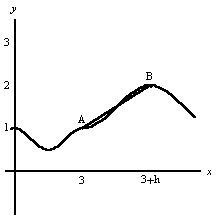The slope of the segment AB is:

 B - A3
 1h
1 h none of those

9.  Which diagram contains the graphs of y = 2x - 2 and  y = -x2 + 2x?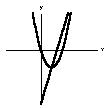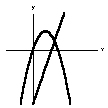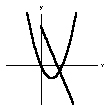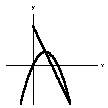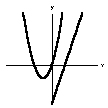10. Identify the graph of x + 1x - 1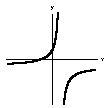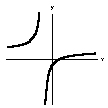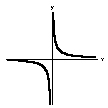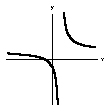11. Find the coordinates of the vertex of the graph of y = x2 + x + 12 .

 (0, 12 )
 ( 12 , 0)
 (- 12 , 12 )
 ( 12 , 14 )
 (- 12 , 14 )

12.  How is the graph of y = x2 - 4x + 3 located relative to the line y = -2?
 It is always above the line It is always below the line It intersects the line at two points The line is tangent to it at one point The line intersects it at one point but is not tangent to it.

13. How far is the point (1, -1) from the center of the circle (x - 2)2 + (y + 3)2 = 2?
Ö2 Ö5
 __Ö13
5 13

14.  find all real values of x such that 16x4 - 81 = 0.

 ± 32
 32
 23
 94
 ± 8116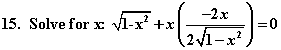± 14
 ± 12
 ±2
 ±Ö2
 ± Ö22

 16.  Solve for w: b(11 + aw) - 44 = b - 13w

 4 - 7b13 + ab
 4 - 7b52 + ab
 15b - 452 + ab
 15b - 413 + ab
 4 - 15b52 + ab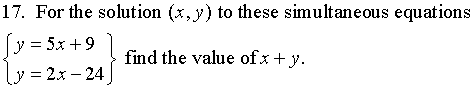-57 -24 9 10 75

18. Given that ab2 = 12 and that ab5 = -96, find a: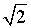-2 -3 84 none of those
 19. Fahrenheit temperature F is obtained from Celsius temperature C by the formula    F = 95 C + 32.

When is the Fahrenheit reading exactly twice the Celsius reading?
 Never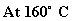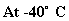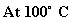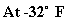Part Two
 20. Let f(x) = x2 + 2x. Find: f(3 + h) - f(3)h ,     assuming h ¹ 0

2h - 9 h - 8 h + 1 8 + h
 h2 + h - 9h

21.  Let f(x) = 10 - 3x. Select the result of subracting f(x) + 1 from f(x+ 1).

 0 10 2f(x + 1) -4 none of those

22.  If g(x) = mx + b, g(4) = -11, and g(-3) = 24, then

 g(x) = -11 g(x) = 24 g(x) = -5x + 9 g(x) = -3x + 24 none of those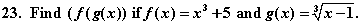x + 4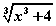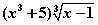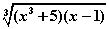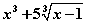24. For the function 1+z2z ,     find all values of z for which f(z) = 2.5

 ± 12
 ±2
2.9
 12 and     2
 - 12 and     -2

25. Let     f(x) =
 x2x - 1 .     Find a formula for f-1(x), the inverse of f.

 2x - 1x
 -x2x - 1
 x2x - 1
 1 - 2xx
x

When used without subscript as in problems 26, 27, and 28, log means log10 and ln means logewith e approximately 2.718.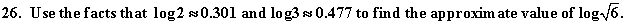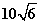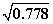0.389 0.072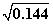27.  Solve for x: 104logx = 9.
 36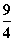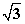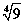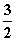28.  Find all x which are solutions to:  log x=2 - log(x -15).

 -5 and 20 5 and 20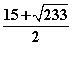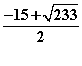20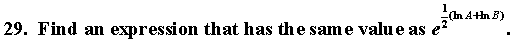12 (A + B )
AB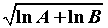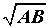eAB

30.  Find x if ln(lnx)=0.
 1 0 e 1/e 10

31.  Solve for x: 49x = 64.
 6449
 ln8ln7
ln64 - ln49 3ln2 - ln7
 ln æçè 6449 ö÷ø

32.  Which of the following is equal to 10?
 ln(10e) 10lne10 eln10 e10 10e

33.  Identify the graph of h(x) = 2x+2 - 8.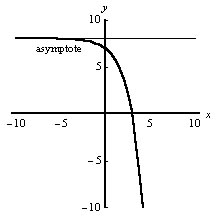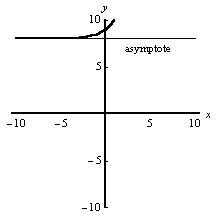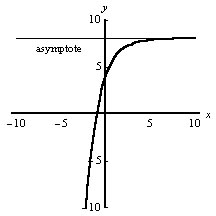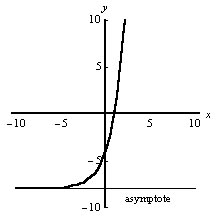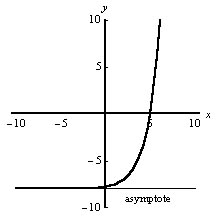34.  Identify the graph of g(x) = ln(x + 1) + 1.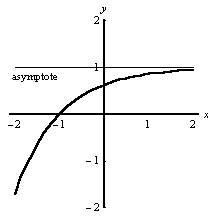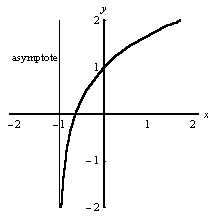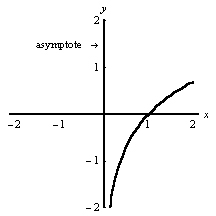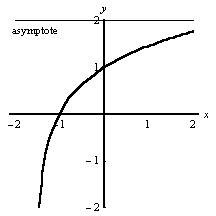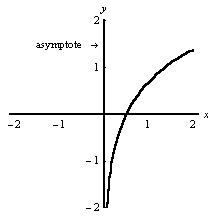Part Three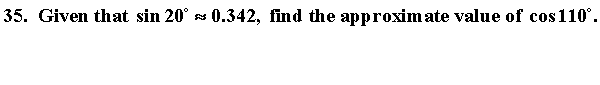-0.94 -0.5 -0.342 0.342 0.94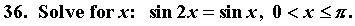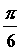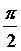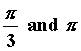No solutions in that interval.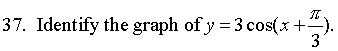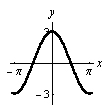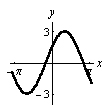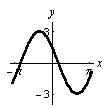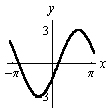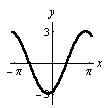38.  Find arccos(-.5) in radians. (Note that arccos means the same as cos-1.)
 p3
 2p3
 - p6
 p6
 - 2p3

 39. The graph of y = 4sin æçè x + p3 ö÷ø crosses the x-axis at x = ?

 2p3
2
 p6
0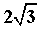40.  Given that cosA = 4/5, find side s.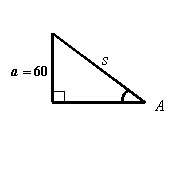36 48 75 80 100

41.  Which of the following equals cos(x + h)?

 cosxcosh + sinxsinh cosxcosh - sinxsinh 2sinxcosh cosxsinh + sinxcosh none of those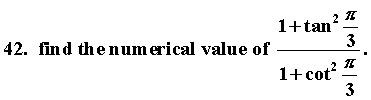9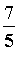1 3 none of those

43.  Find x, the length of the indicated side.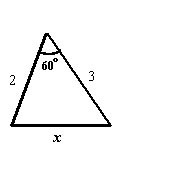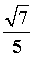2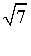3